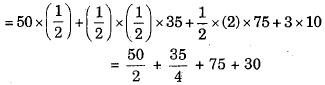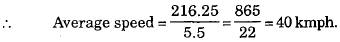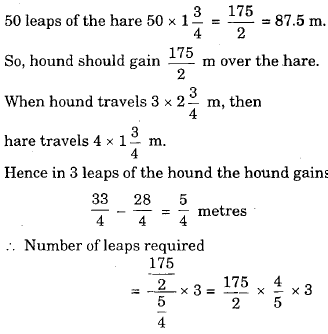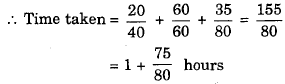# Engineering Maths - Time and Work

>>>>>>>>Time and Work

Refer to the data below and answer the questions that follow.
The variation in the speed of a car on a particular day at the respective times is shown in the table below:

 s(km/hr) 0 40 50 85 10 10 t(hr) 11.00 am 11.30 am 1.00 pm 1.30 pm 3.30 pm 4.30 pm

• A

6.30 p.m.• B

8.45 a.m.• C

10.30 p.m.• D

12.15 a.m.• Option : D
• Explanation : Distance covered are the same.
Speed in the latter is maintained at 10 kmph.
∴ 77.5 = 10 × x
=> x= 7.75 hours.
Hence, clock time will be 4.30 + 7 hours 45 mins
= 12.15 a.m.

Refer to the data below and answer the questions that follow.
The variation in the speed of a car on a particular day at the respective times is shown in the table below:

 s(km/hr) 0 40 50 85 10 10 t(hr) 11.00 am 11.30 am 1.00 pm 1.30 pm 3.30 pm 4.30 pm

• A

22.1 kmph• B

54.3 kmph• C

39.3 kmph• D

86.5 kmph• Option : C
• Explanation :Distance travelled from 1.00 pm to 4.30 p.m.
= A(ΔBQRY) + A(ΔBYC) + A(ΔCZD) + A(ΔZRTE)= 130 + 8.75
= 138.75 km.
Total distance travelled = 77.5 + 138.75
= 216.25 km.• A

300• B

200• C

150• D

250• Option : A
• Explanation :
While deer makes 10 leaps, cheetah makes 6 leaps.
∴ Distance covered in 10 leaps by deer
= 10 x 1 = 10m
Distance covered in 6 h~aps by cheetah
= 6 x 2 = 12 m
Hence in six leaps, cheetah gains
12 - 10 = 2 m over the deer.
Cheetah has to gain 100 m over the deer.
So, total leaps required by cheetah
= 6/2 x 100 = 300

• A

70 leaps• B

36 leaps• C

210 leaps• D

328 leaps• Option : C
• Explanation := 210 leaps

Refer to the graph below and answer the questions that follow.
The engine of the new car Palio brought by Fiat in the market follows certain mileage characteristics as shown in the diagram below:Vijay bought the new car Palio and filled exactly 10 litres in his car. He goes at a speed of 40 km/hr for first 20 km and then at a speed of 60 km/hr for next 60 km and driving at a constant speed reaches his destination which was 115 km away from his starting point.

• Option : C
• Explanation :
Fuel required for first 20 km = 20/8 = 2.5 litres
Fuel required for next 60 km = 60/15 = 4 litres.
∴ Petrol left = 10 - 6.5 = 3.5 litres.
Now, he has to travel = 115 - (60 + 20)
= 35 km in 3.5 litres.
So he can go at a speed of either 45 km/hr or 80 km/hr as mileage is 10 km/litre in both cases. But since he reaches in minimum time, he travels at 80 km/hr= 1 hours 56 minutes and 25 seconds.
Related Quiz.
Time and Work